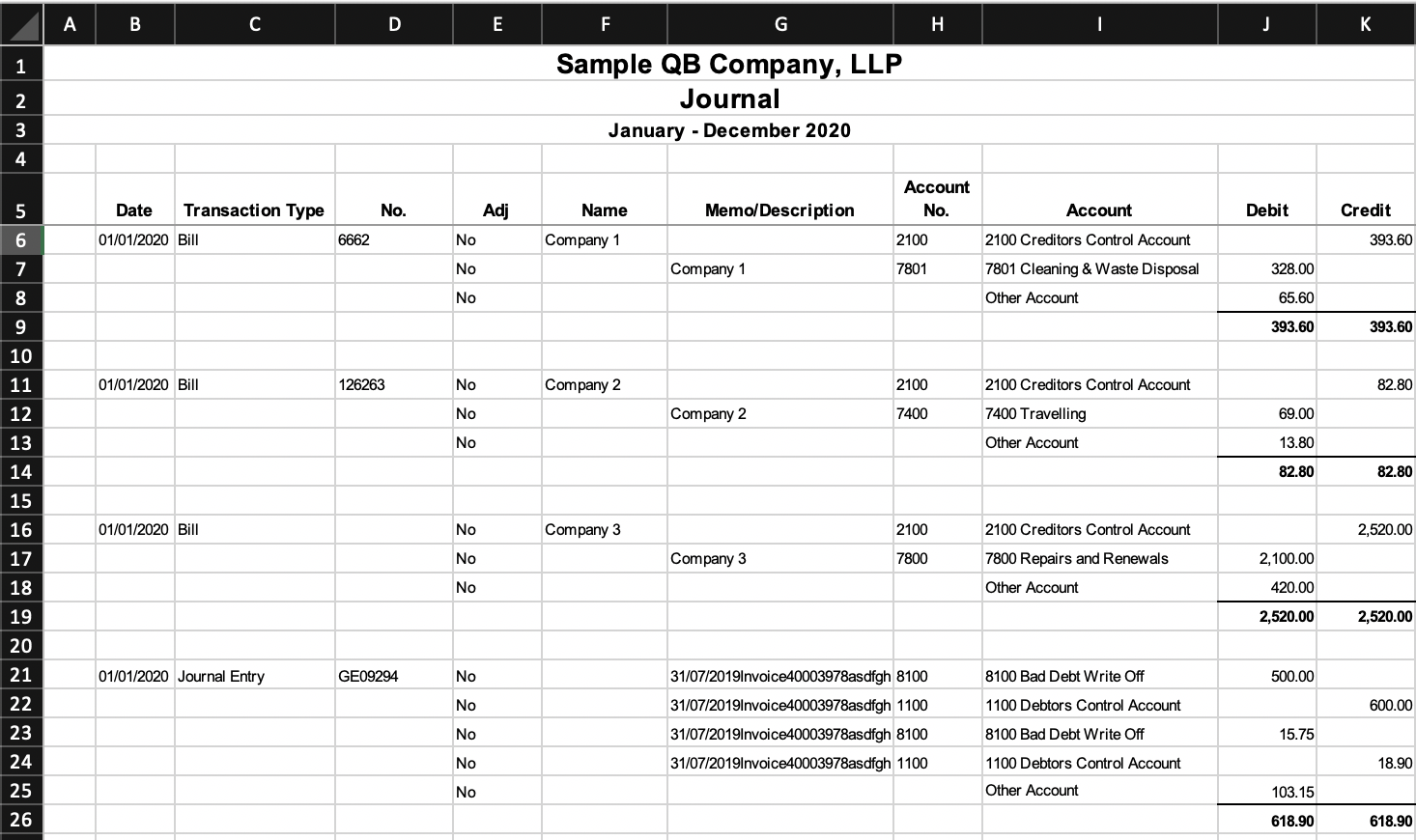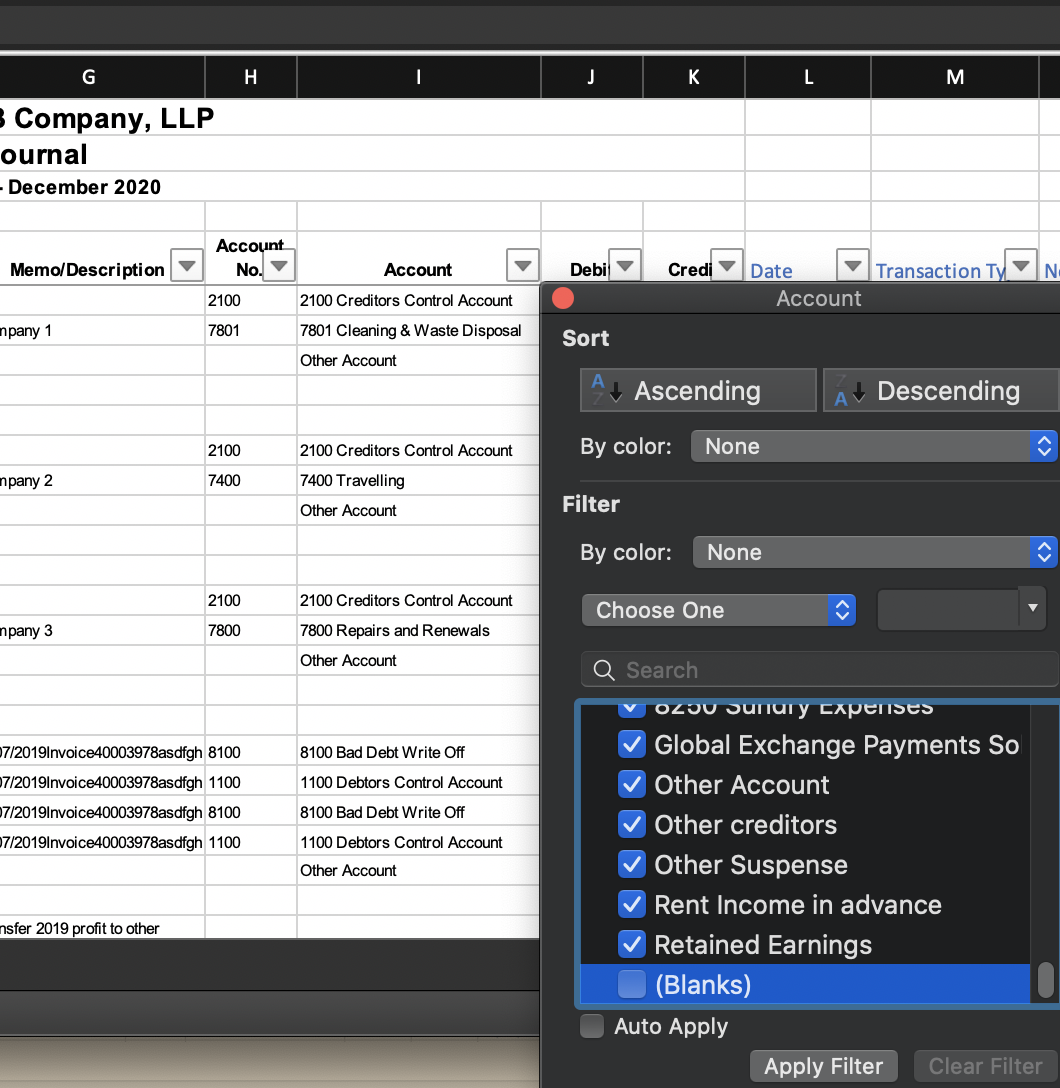# Data Formatting Guide: Simple degrouping of multiple columns, fill in blank account numbers (common QuickBooks example)

• Updated

## Summary

Learn how to ungroup a data file by filling down multiple columns to produce a file that is supported by MindBridge. This is a common problem in many data exports taken from bookkeeping systems such as QuickBooks.

This method can even be used if not all columns have a value on the first row of the group, and to fill in blank account numbers when the account description is present but the account number is not.

Note: If possible, first try to set up a direct connector or have your client connect to pull the data directly into MindBridge, or follow these instructions to export from QuickBooks Online or QuickBooks Desktop in order to avoid needing to ungroup the data at all. This guide is meant for situations in which it is impossible to set up a direct connector or obtain a better export.

## Resolution

Consider the following excerpt from a QuickBooks General Ledger file:### The problems

1. This file is grouped by transaction; Date, Transaction Type, No., and Name are present on only the first row in the group of transactions and need to be dragged down.
2. Account No. is not always present (see for example rows 8, 13, 17, 25), but Account is. Account can be mapped to Account ID, but Account No. cannot unless the blanks are filled in.
3. There are unneeded transaction subtotal rows (9, 14, 19, 26)

### The solution (formatting needed)

1. Drag Date, Transaction Type, No., and Name down to each transaction row
2. Fill in the blank Account No. with the value in Account
3. Exclude extraneous total/subtotal/blank rows (9, 14, 19, 26) and extraneous rows (rows 1-4) above the header row (row 5)

We will put the revised columns at the end of the file.

• Date and Transaction Type are always present on the first row of the transaction group, but No. and Name are sometimes blank for a group. See the 3rd and 4th transaction groups beginning on rows 16 and 21.
• Our logic will be to take the value from the original column if we are on the first row of the transaction, or the previous value we found if we are not on the first row of the transaction.

### Revised Date: Cell L6 and below`=IF(LEN(\$B6)>0,IF(LEN(B6)>0,B6,""),L5)`
• `LEN(\$B6)>0`
tests if there is a value in column B by testing if the length is greater than 0. Since as we observed, Date is always present on the first row of the transaction group, this serves as our check if we are on the first row of the transaction. We put \$ in front of the B for ease of copying the formula to the rest of the columns to drag down (Transaction Type, No., Name), as we will always be checking the presence of Date to check if we are on the first row of the transaction
• `IF(LEN(B6)>0,B6,"")`
returns the date if it is present, "" (empty string) if it is not. This can be simplified to simply B6 for the Date column because the Date is always present on the first row of the transaction group, but this logic is used to be able to copy and paste the same formulas for No. and Name, which, as noted above, are not always present. Because Excel will display 0 instead of blank when referencing an empty cell, we have to tell it to return "" in order for it to display an empty cell.

Note: The above formulas checking for empty string assume that the cells that look empty are indeed empty in Excel. If these empty-looking cells actually contain spaces, non-breaking spaces, or phantom data, substitute the following for all instances of B6 within the LEN() function:

`TRIM(CLEAN(SUBSTITUTE(B6,CHAR(160)," ")))`
• The TRIM() removes spaces
• CHAR(160) indicates the non-breaking space character, and the SUBSTITUTE() formula around it removes the non-breaking space characters (note: on a Mac, use CHAR(202) instead of CHAR(160))

So the final formula would be:

`=IF(LEN(TRIM(CLEAN(SUBSTITUTE(\$B6,CHAR(160)," "))))>0,IF(LEN(TRIM(CLEAN(SUBSTITUTE(B6,CHAR(160)," "))))>0,B6,""),L5)`

### Revised Transaction Type, No., Name

Copy and paste and drag down the formula found for the revised Date.The formulas in cells M6, N6, and O6 should be the following:

`=IF(LEN(\$B6)>0,IF(LEN(C6)>0,C6,""),M5)=IF(LEN(\$B6)>0,IF(LEN(D6)>0,D6,""),N5)=IF(LEN(\$B6)>0,IF(LEN(F6)>0,F6,""),O5)`

### Revised Account No.

Our logic to fill in the blanks for Account No. in column P will be to take the original Account No. from column H if it is present (length is greater than 0), Account from column I if it is not (length is not greater than 0):`=IF(LEN(H6)>0,H6,I6)`

### Filter to keep only transaction rows

We observe that every transaction row in the original file has an Account. So with filters turned on, filter out blanks from column I (Account):### Copy and paste values into a new workbook

Then select the header row and selected transaction rows (i.e., row 5 onward in the filtered view - you can start at cell B5, hit Ctrl-Shift-End to get to the end of the file, and adjust the position as necessary), then copy, then paste special values in a new workbook.

Anything else on your mind? Chat with us or submit a request for further assistance.